Function Repository Resource:

# QuantumTensorAutomaton

A quantum cellular automaton model that evolves the tensor product of a collection of initial qubits using arbitrary compositions of unitary operators for a finite number of steps

Contributed by: Ruhi Shah and Jonathan Gorard
 ResourceFunction["QuantumTensorAutomaton"][matrices,initial,t] evolves initial for t steps using a composition of unitary matrices given by matrices.

## Details and Options

Any number of unitary matrices can be composed. The argument matrices must be a list.
Use "ColourPlot"True to visualize the state vectors of the individual steps.
Setting "Norms"True shows the norm-squared probability of being in a given state.
WIth "PlotNorms"True, ResourceFunction["QuantumTensorAutomaton"] shows the plot of norm-squared probabilities over time.
Common operators to use in the first argument include:Rotation: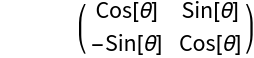CNOT:Pauli (X/Y/Z):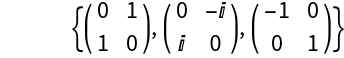Square root of NOT:Fourier:Any unitary operator

## Examples

### Basic Examples (5)

Define a "CNOT" operator:

 In:=Apply the operator "CNOT" 10 times to an initial state:

 In:=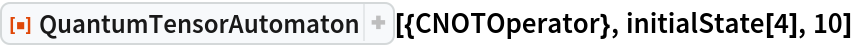Out=Visualize the state vectors for the preceding:

 In:=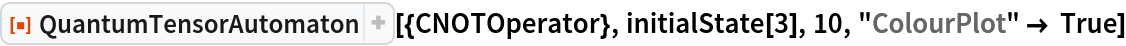Out=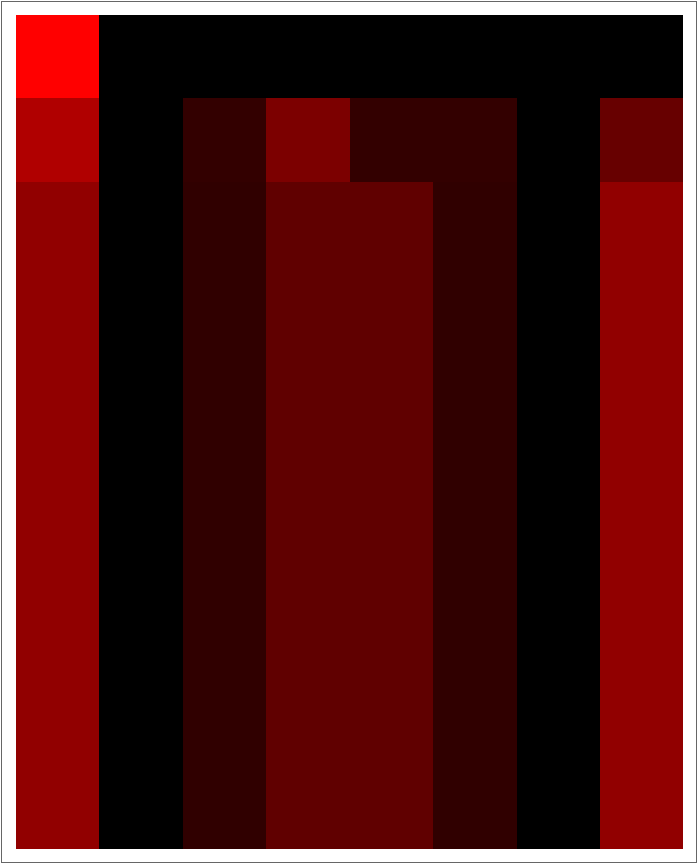Compute the probabilities (norms squared) for the preceding:

 In:=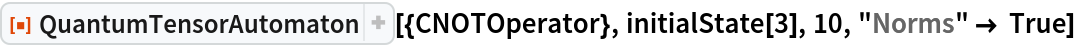Out=Plot the probabilities (norms squared) against the steps (time) for the preceding:

 In:=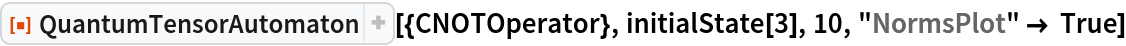Out=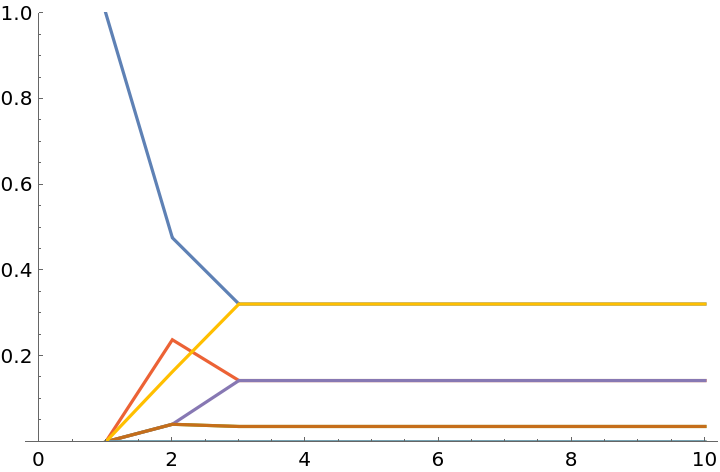### Scope (2)

Define and compose multiple operators:

 In:=Out=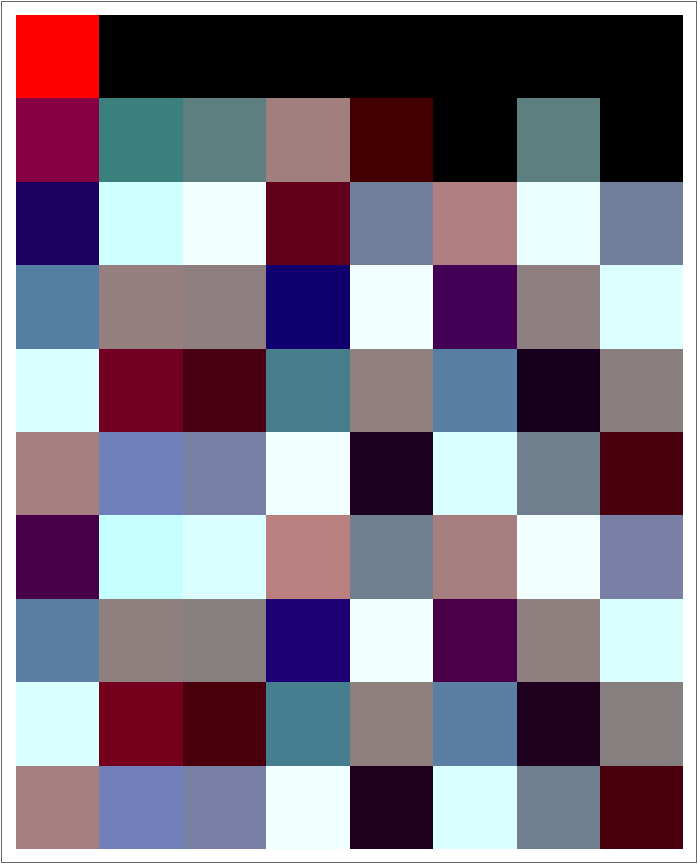Random unitary operators will also work:

 In:=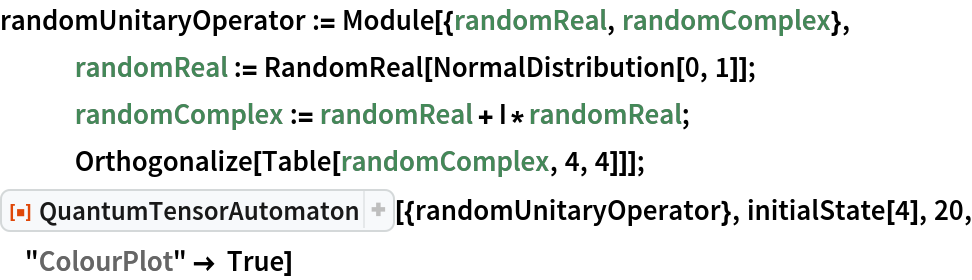Out=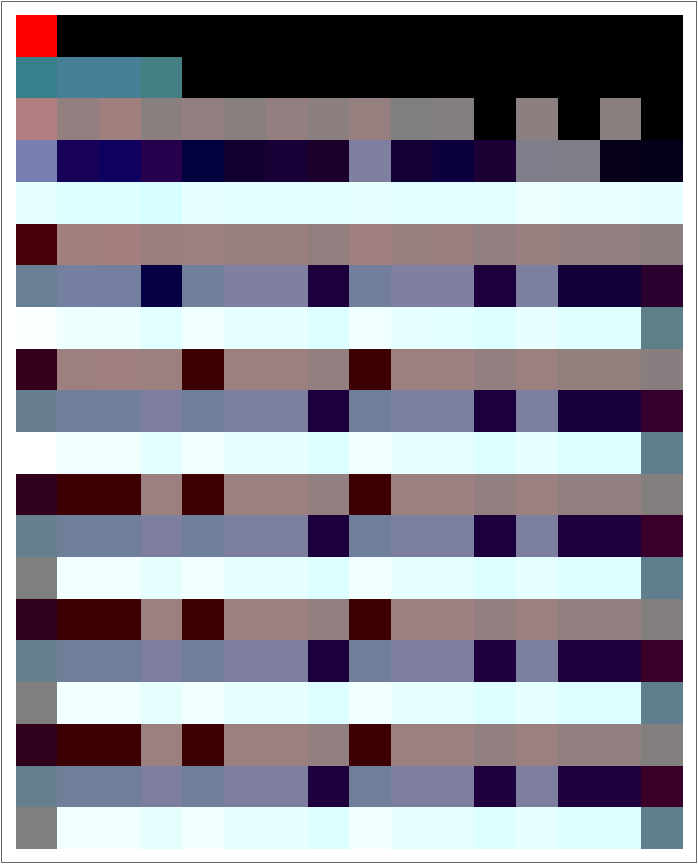### Neat Examples (3)

#### Resonance  (3)

Some operators have unique resonance properties:

 In:=Out=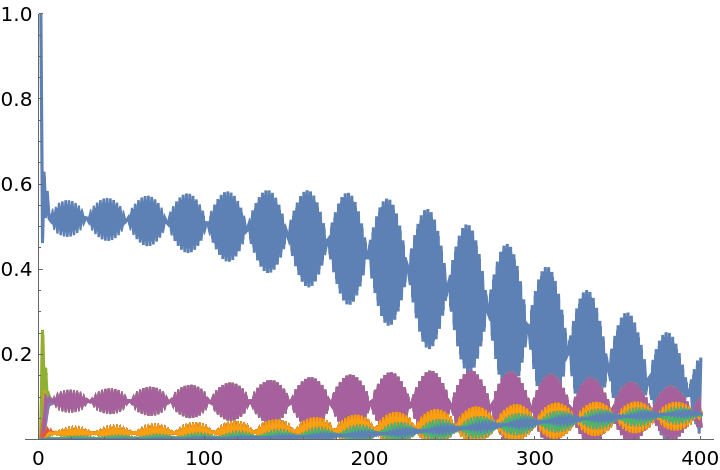This can be visualized in Fourier space:

 In:=Out=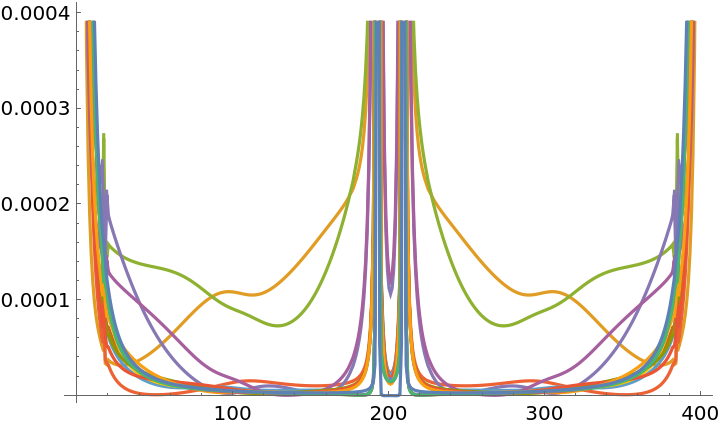reveals the main frequencies:

 In:=Out=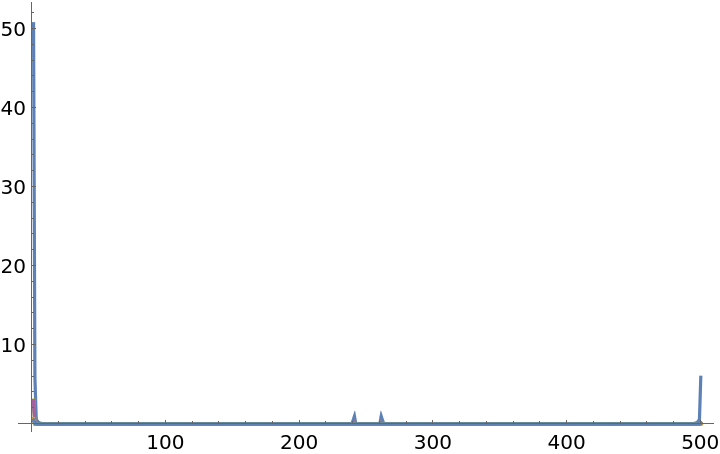Jonathan Gorard

## Version History

• 1.0.0 – 06 August 2019